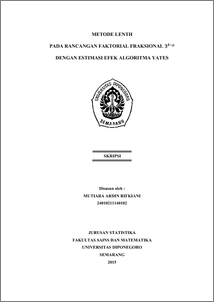# METODE LENTH PADA RANCANGAN FAKTORIAL FRAKSIONAL 3^(k-p) DENGAN ESTIMASI EFEK ALGORITMA YATES

RIFKIANI, MUTIARA ARDIN (2015) METODE LENTH PADA RANCANGAN FAKTORIAL FRAKSIONAL 3^(k-p) DENGAN ESTIMASI EFEK ALGORITMA YATES. Undergraduate thesis, FSM Universitas Diponegoro.Preview
PDF
7Mb

## Abstract

Factorial design often is used in experiments on various fields to identify the influence of main factors and interaction factors to respons were observed. A design which has k factors with three levels for each factor called 3^k factorial design. For a large number of factors, fractional factorial design 3^(k-p) is an effective alternative because it has less combination of treatment than 3^k factorial design, but it still has important needed information. In experiments conducted without repetition, determining factors that influence towards response is difficult to be analyzed if using analysis of variance. It was due to the the average of squared error absence, where error variance estimation is based on the variability of the data obtained from repeated observations. To overcome this, we use Lenth Method to identify the factors that affect the response. Lenth method uses the value of the statistic margin of error (ME) test for the main factor, and simultaneous margin of error (SME) for the interaction factor. The calculation of the statistic test ME and SME values are based on the estimated effects of each treatment. Yates algorithm is used to calculate the effect’s estimation for each treatment. To clarify the discussion about this matery is given an example of fractional factorial design 3^(4-1) application with 27 experiments on combustion boiler. The results indicate that treatment factors are influenced towards the response are D, ABCD^2,ABC,〖AB〗^2, AC^2 D^2, BC^2 D^2 dan B^2 CD. Keywords: three-level fractional factorial, factorial without replication, Lenth Methods, Yates Algorithm

Item Type: Thesis (Undergraduate) H Social Sciences > HA Statistics Faculty of Science and Mathematics > Department of Statistics 47338 Mr Hasbi Yasin 11 Jan 2016 15:08 11 Jan 2016 15:08

Repository Staff Only: item control page